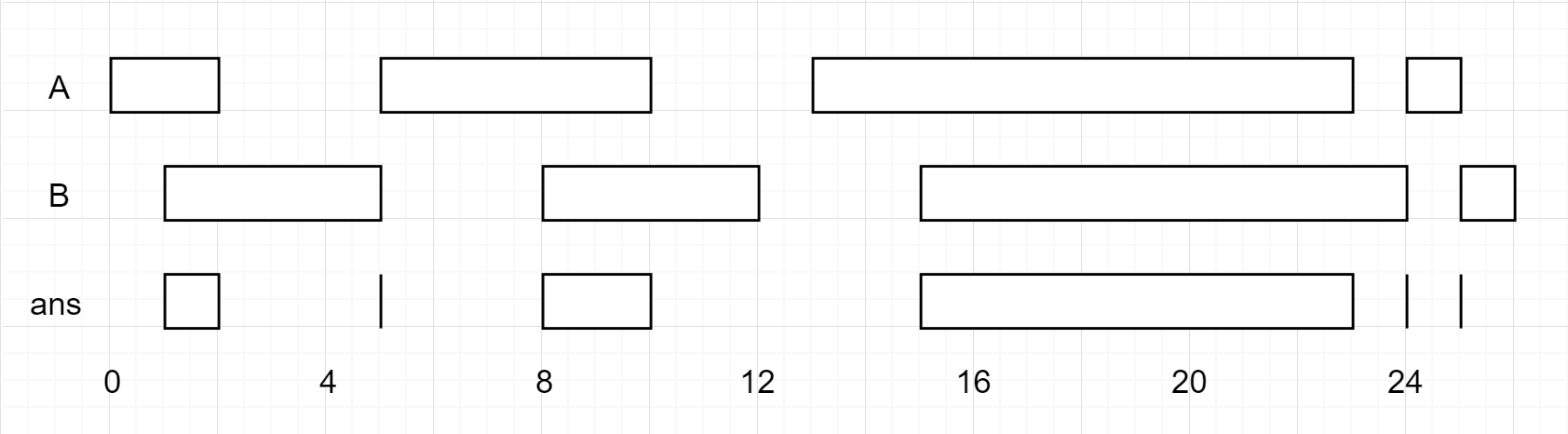## 986. Interval List Intersections

You are given two lists of closed intervals, `firstList` and `secondList`, where `firstList[i] = [starti, endi]` and `secondList[j] = [startj, endj]`. Each list of intervals is pairwise disjoint and in sorted order.

Return the intersection of these two interval lists.

A closed interval `[a, b]` (with `a < b`) denotes the set of real numbers `x` with `a <= x <= b`.

The intersection of two closed intervals is a set of real numbers that are either empty or represented as a closed interval. For example, the intersection of `[1, 3]` and `[2, 4]` is `[2, 3]`.

Example 1:```Input: firstList = [[0,2],[5,10],[13,23],[24,25]], secondList = [[1,5],[8,12],[15,24],[25,26]]
Output: [[1,2],[5,5],[8,10],[15,23],[24,24],[25,25]]
```

Example 2:

```Input: firstList = [[1,3],[5,9]], secondList = []
Output: []
```

Example 3:

```Input: firstList = [], secondList = [[4,8],[10,12]]
Output: []
```

Example 4:

```Input: firstList = [[1,7]], secondList = [[3,10]]
Output: [[3,7]]
```

Constraints:

• `0 <= firstList.length, secondList.length <= 1000`
• `firstList.length + secondList.length >= 1`
• `0 <= starti < endi <= 109`
• `endi < starti+1`
• `0 <= startj < endj <= 109 `
• `endj < startj+1`

## Rust Solution

``````struct Solution;

impl Solution {
fn interval_intersection(a: Vec<Vec<i32>>, b: Vec<Vec<i32>>) -> Vec<Vec<i32>> {
let mut res: Vec<Vec<i32>> = vec![];
let mut i: usize = 0;
let mut j: usize = 0;
let n = a.len();
let m = b.len();
while i < n && j < m {
let al = a[i];
let ar = a[i];
let bl = b[j];
let br = b[j];
if ar < bl {
i += 1;
continue;
}
if br < al {
j += 1;
continue;
}
let l = i32::max(al, bl);
let r = i32::min(ar, br);
res.push(vec![l, r]);
if ar < br {
i += 1;
} else {
j += 1;
}
}
res
}
}

#[test]
fn test() {
let a: Vec<Vec<i32>> = vec_vec_i32![[0, 2], [5, 10], [13, 23], [24, 25]];
let b: Vec<Vec<i32>> = vec_vec_i32![[1, 5], [8, 12], [15, 24], [25, 26]];
let res: Vec<Vec<i32>> = vec_vec_i32![[1, 2], [5, 5], [8, 10], [15, 23], [24, 24], [25, 25]];
assert_eq!(Solution::interval_intersection(a, b), res);
}
``````

Having problems with this solution? Click here to submit an issue on github.